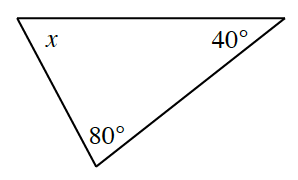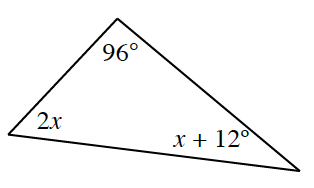### Home > CCAA > Chapter 9 Unit 10 > Lesson CC3: 9.1.4 > Problem9-49

9-49.

Use what you know about the angles in a triangle to find $x$ in each diagram below. Show all work. Then classify each triangle as acute, right, or obtuse.

By the Angle Sum Theorem, the sum of all interior angles of a triangle is always $180º$.

1.$x + 40 + 80 = 180$

$x + 120 = 180$

$x = 60°$

1.$(2x) + (x + 12) + (96) = 180$